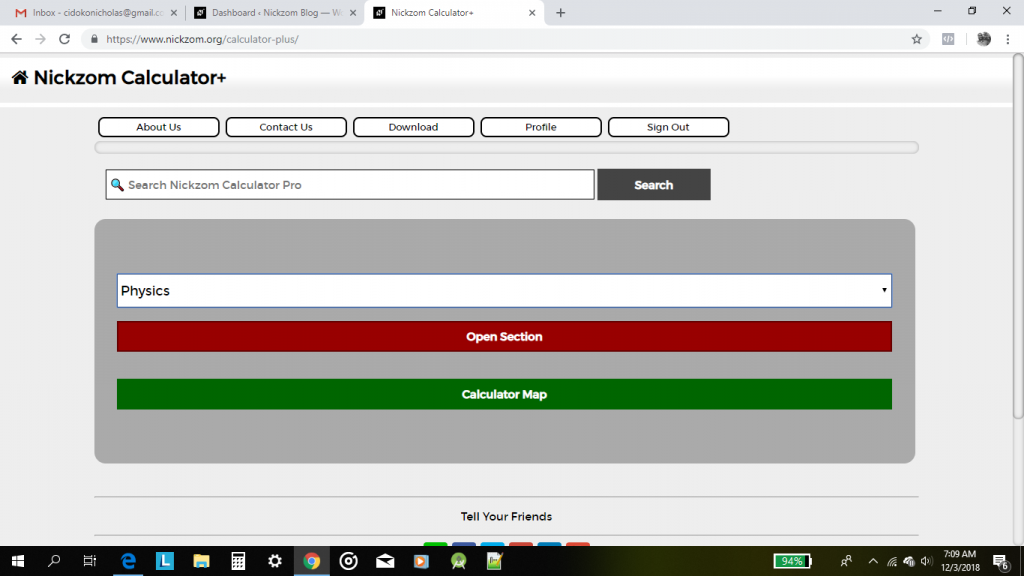## Nickzom Calculator Calculates Range and Maximum Range of a Projectile in Physics

To get the answer for the range of a projectile, there are three essential parameters to be given which are:

• Acceleration due to Gravity
• Initial Velocity
• Angle of Projection

Where as to get the answer for maximum range of a projectile requires two parameters to be given which are:

• Acceleration due to Gravity
• Initial Velocity

Let’s first, proceed to the home page of the app. Select Physics and click on Open SectionThen proceed to click on Projectile